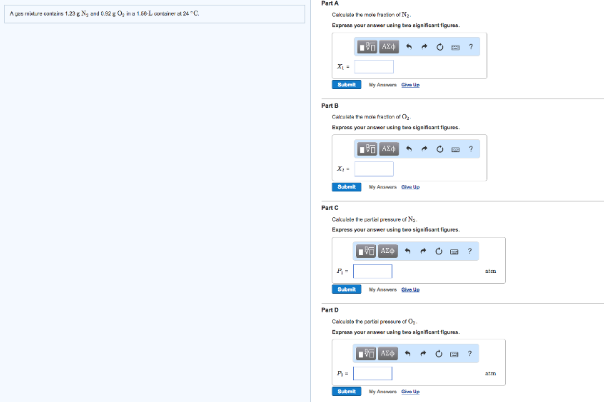# Problem: A gas mixture contains 1.23 g N2 and 0.92 g O2 in a 1.68-L container at 24°C. Calculate the mole fraction of N2. Express your answer using two significant figures. Calculate the mole fraction of O2. Express your answer using two significant figures. Calculate the partial pressure of N2. Express your answer using two significant figures. Calculate the partial pressure of O2. Express your answer using two significant figures.

###### FREE Expert Solution###### Problem Details

A gas mixture contains 1.23 g N2 and 0.92 g O2 in a 1.68-L container at 24°C.

Calculate the mole fraction of N2. Express your answer using two significant figures.

Calculate the mole fraction of O2. Express your answer using two significant figures.

Calculate the partial pressure of N2. Express your answer using two significant figures.

Calculate the partial pressure of O2. Express your answer using two significant figures.